## 4.4 Complex plots

The commands covered so far provide shortcuts and all do multiple things (e.g., choose default layouts, select value range and labels on axes, as well as drawing particular objects). To gain more control about the details of our plots or modify some of the default choices, we need to explicitly specify all parts of a plot.

Complex plots are created by multiple function calls and typically start by calling plot() with type = "n" to create a basic plot or canvass, before adding additional objects to it. More specific plotting functions include grid(), abline(), points(), text(), title(). As these functions add specific elements to an existing plot, they are also known as annotation functions. By setting graphical parameters (with the par() function), we can influence the overall appearance and arrangement of plots.

Given the long history of R and the graphics and grDevices packages that contain its visualization functions, we can only cover a selection here. In the following, we will sketch the general workflow of complex graphics and illustrate some important functions in the context of some visualizations.

### 4.4.1 Composing plots as programs

More advanced plots in the base R plotting system are created by calling successive R functions to incrementally build a visualization.

When constructing more advanced visualizations, plotting occurs in two main stages:

1. Calling plot() for creating and defining the dimensions of a new plot;

2. Using annotation functions for adding elements to the plot (e.g., points, lines, or text elements, as well as grids, titles, labels, and legends).

This two-stage process essentially turns the holistic task of “making a plot” into one of step-wise planning and composition. Rather than just calling one command that creates the entire plot, we start with a simple and reduced version (think: a blank canvas) and then use the tools provided by R (think: brushes, color, shapes, etc.) to improve our visualization.

Actually, creating beautiful and convincing visualizations typically requires an additional step. Whenever we want to change the standard values that govern the properties or appearance of a plot, we may have to adjust the default graphical parameters or define some auxiliary objects to be used in the plot (e.g., a palette of colors). If we include the preparatory steps that precede plotting, the process of creating a complex visualization is structured like any other computer program:

1. Define data objects and other elements to be used (e.g., the values of titels, colors) or change existing graphical parameters (e.g., how plots are to be arranged on the canvas);

2. Prepare the plotting canvas (e.g., its dimensions, axes);

3. Add objects by calling functions that create visual elements (e.g., points, lines, labels, titles, etc.).

Note that — just as in other programs — the order of operations matters. When creating complex plots, this is true in both a logical and a visual sense: We generally need to define an object (e.g., a value or color) prior to using it in an evaluated expression or function. But when creating a visualization and wanting to show some object A in front of another object B, we need to draw B before drawing A (so that A is plotted later than/on top of B).

### 4.4.2 Starting a new plot

We have seen above that the plot() function can create a scatterplot, or other types of plot depending on the type of object being plotted. However, plot() can also be used to merely create a blank plot (i.e., start a new screen device, e.g., in the Plots window of RStudio) to which we then add the elements of our graph.

To understand this process, evaluate and compare the result of the following commands:

plot(x = 0)
plot(x = 0, type = "n")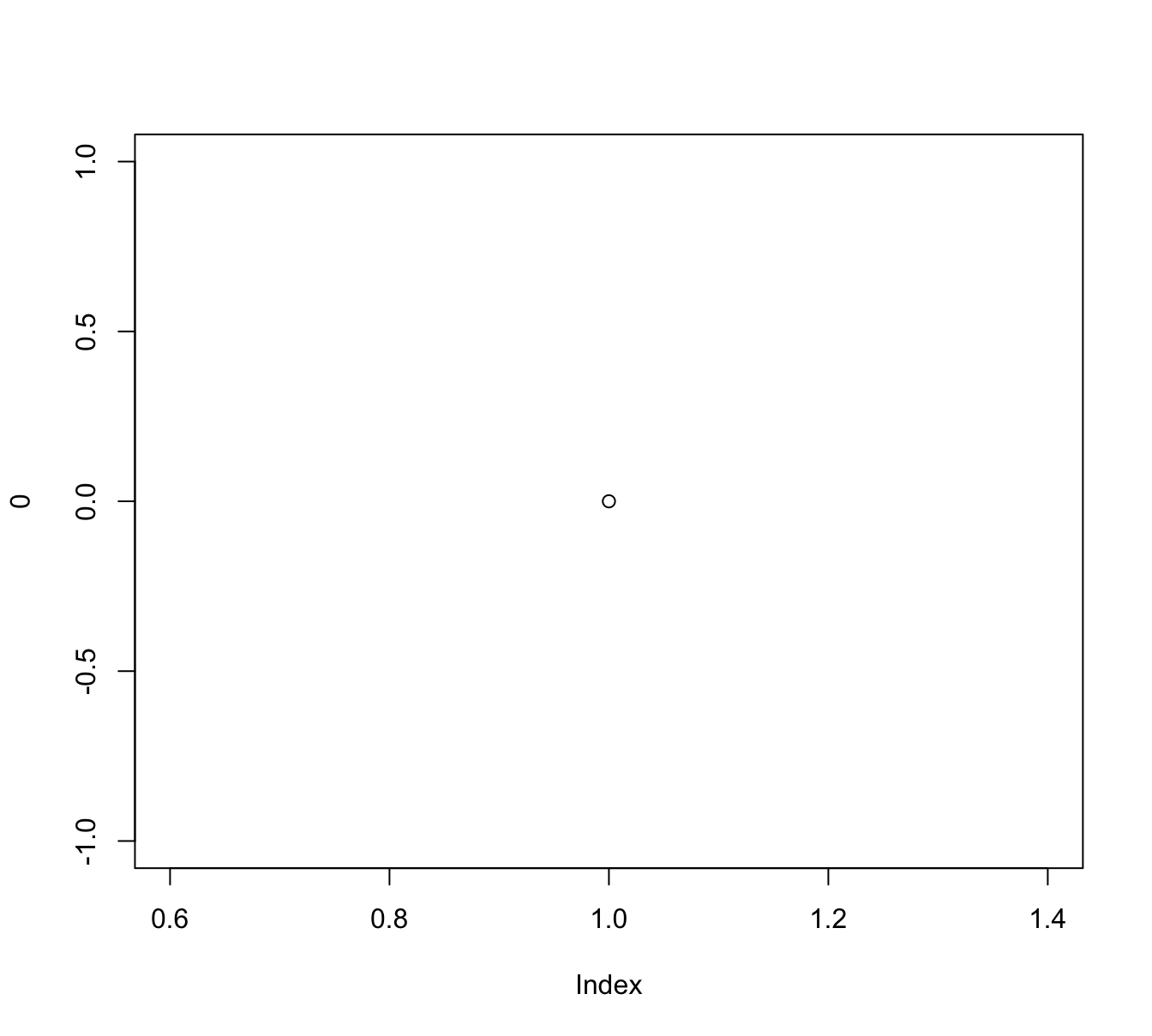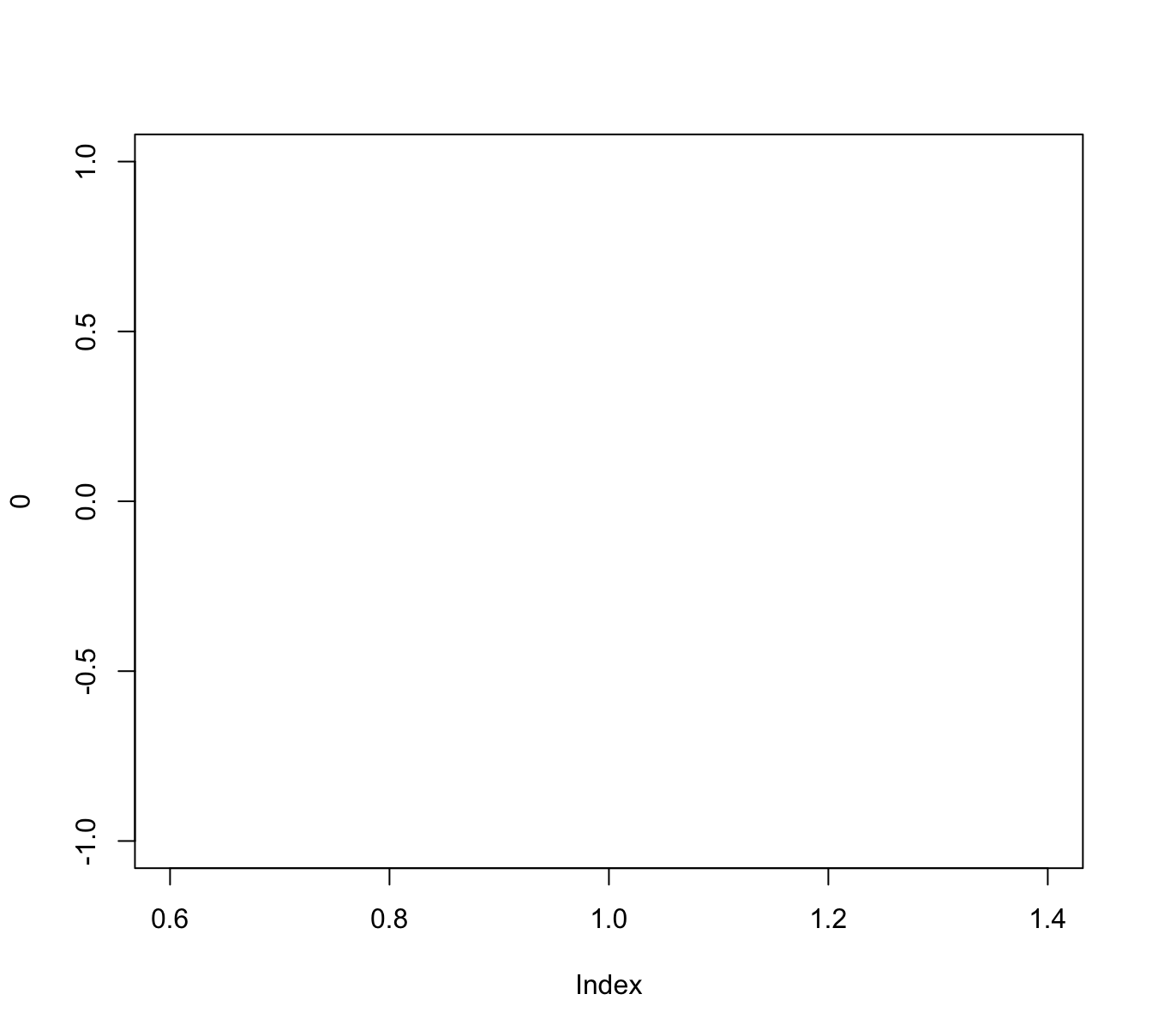We see that both commands create a new plot, but plot(x = 0) creates a scatterplot of a single point (with coordinates of $$(0, 0)$$) whereas adding the argument type = "n" instructed R to merely define a new plotting canvas. However, the new plot still used x to choose ranges for both axes. If we want different ranges, we either can change our data inputs (to x and/or y) or explicitly control the ranges of axes:

# (a) use data to define ranges:
xs <- -10:10
ys <- xs^2

plot(x = xs, y = ys, type = "n")

# (b) define ranges without data:
plot(x = 0, xlim = c(-10, 10), ylim = c(0, 100),  type = "n")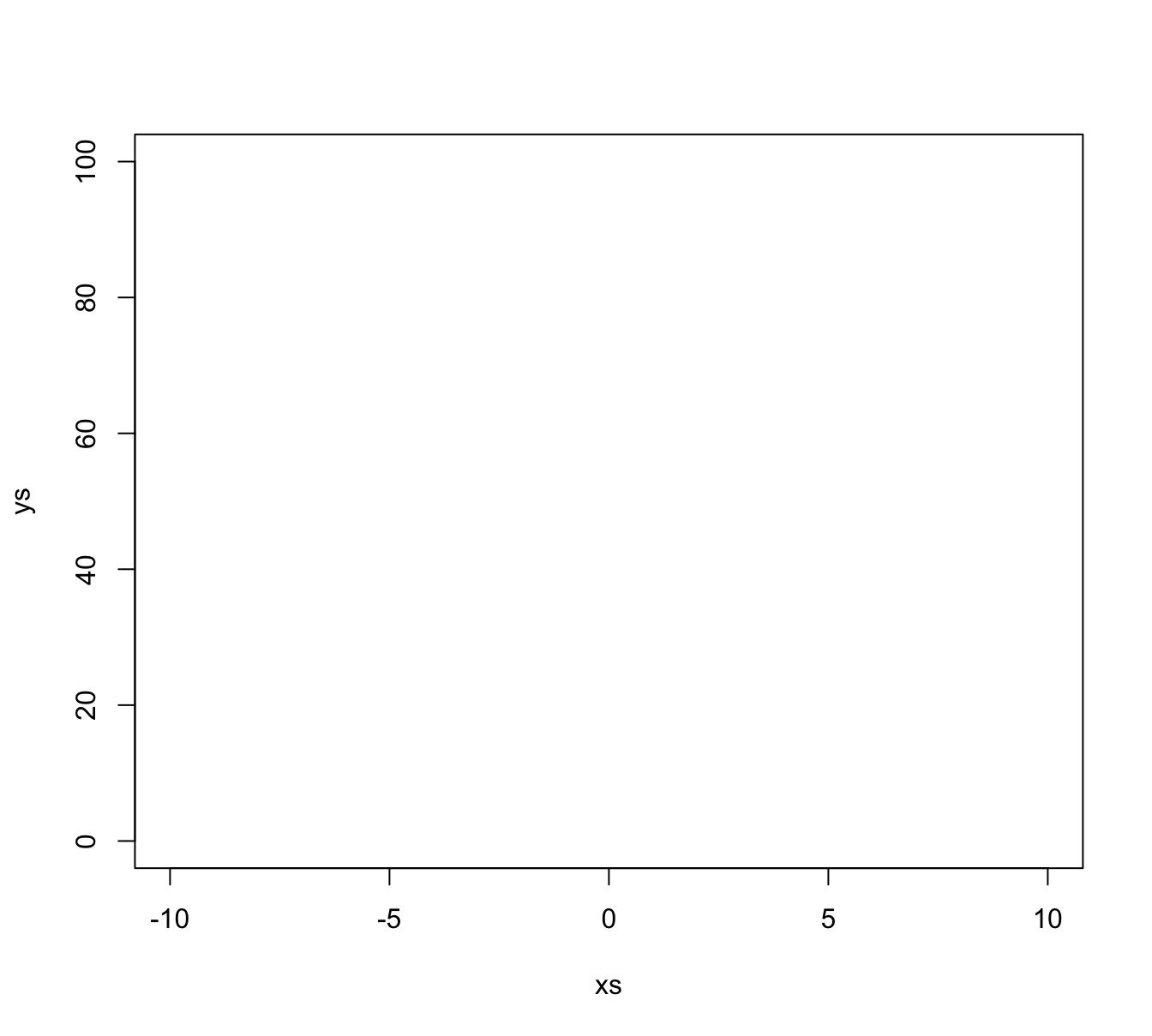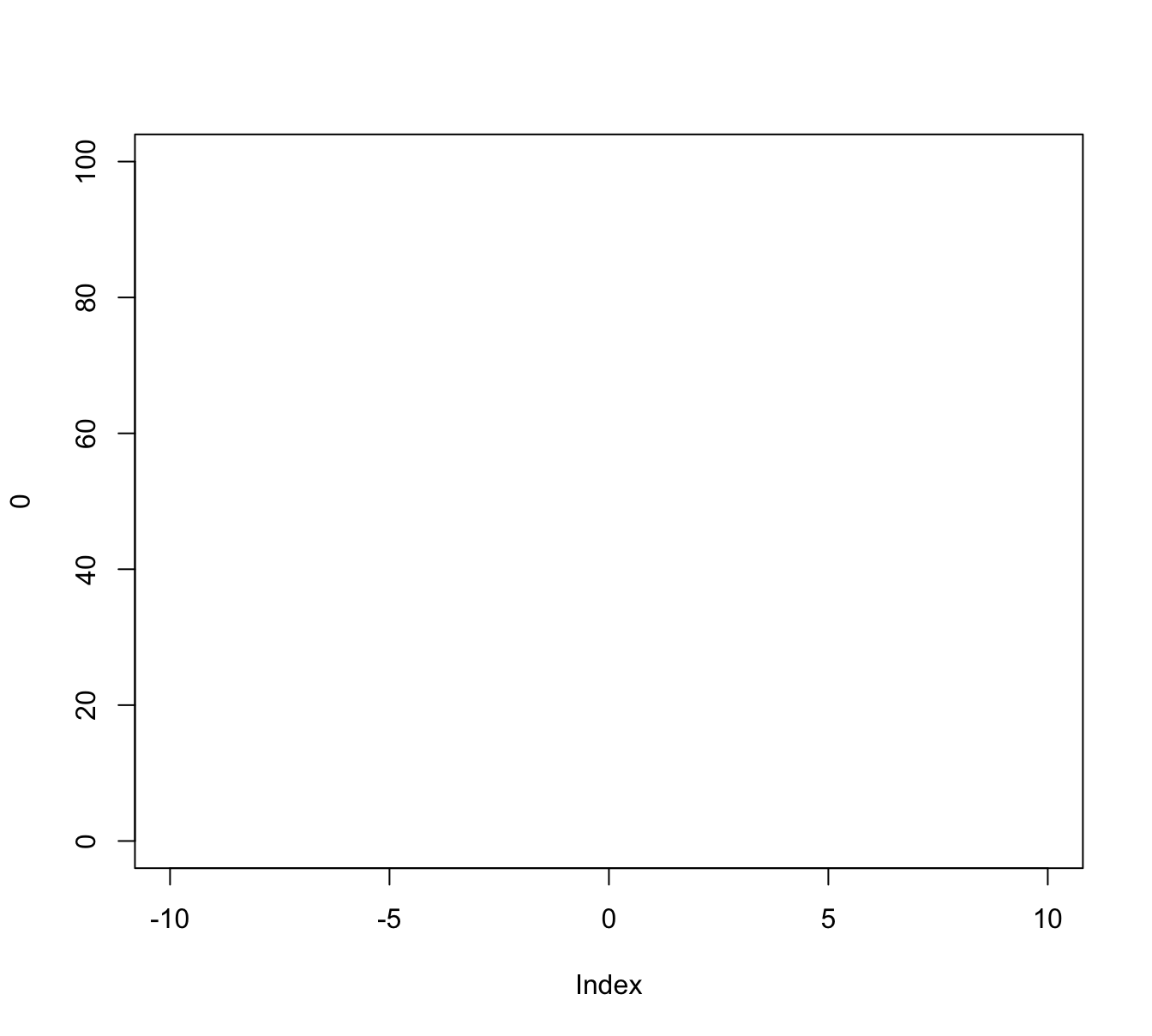Note that both plots just created use the same ranges, but differ in the labels used on the x- and y-axes. If we wanted to control these manually, we could have used the xlab and ylab arguments of plot().

### 4.4.3 Annotating plots

Once we have created a new screen device and defined the dimensions of our plotting region, we can use so-called annotation functions to add to an existing plot. Some key annotation functions include:

• points() adds points (or circles) to a plot

• abline(), lines(), and segments() add various types of lines to a plot (given their  x and y coordinates, or defining other properties, like a linear function’s intercept and slope)

• rect() adds rectangles or boxes (given the coordinates of their corners)

• text() adds text labels to a plot (to specific x and y coordinates)

Other functions allow adding or editing elements on the plot background, axes, and margins:

• axis() adds axis labels and ticks

• grid() adds orientation lines

• title() adds labels to the axes, title, and subtitle, and outer margin

• mtext(): add arbitrary text labels to the plot’s (inner or outer) margins

Overall, these and other functions allow the composition of arbitrarily complex visualizations. As an example, the following code illustrates the process of first defining a plotting region and then using functions for adding various points, lines, shapes, and text elements. To really understand this process, it makes sense to evaluate the chunk in a step-by-step fashion (i.e., one command at a time):

# (0) Define some colors: -----
library(unikn)  # to get color palettes
my_colors <- usecol(pal_unikn_light)
# seecol(my_colors)  # view color palette

# (1) Create canvas (but specify dimensions and some labels): -----
plot(x = 0, xlim = c(-10, 10), ylim = c(0, 100),
type = "n",
main = "Plotting various shapes and text labels",
xlab = "X value", ylab = "Y value")

abline(h = 50, lty = 2)
abline(v =  0, lty = 2)
abline(a = 10, b = 10)

# (3) Add points (as circles): -----
points(x = c(-5, 0, 5), y = c(40, 50, 60),
pch = 21, cex = 20, lwd = 4,
col = my_colors[c(1, 3, 5)],
bg  = usecol("grey", alpha = 1/2))

# (4) Add text labels: -----
text(x =  0, y = 50, labels = "R", cex = 4)
text(x = -5, y = 40, labels = "my", cex = 2)
text(x =  5, y = 60, labels = "stuff", cex = 2)

# (5) Add other shapes: -----
rect(xleft = c(-1, -9), xright = c(7, 1),
ybottom = c(0, 80), ytop = c(20, 95),
col = usecol(my_colors, alpha = 1),
border = my_colors[c(7, 5)], lwd = 4)

# (6) More text labels: -----
text(x =  -4, y = 87, labels = "R pour l'art", col = Bordeaux, cex = 1.5)
text(x =  3, y = 10, labels = "WOW!", col = Karpfenblau, cex = 3)

# (7) Add line segments: -----
segments(x0 = c(-4, -4, 5, 9), y0 = c(20, 40, 25, 30),
x1 = c(9, 3, -8, -4), y1 = c(35, 85, 60, 80),
lwd = 5, col = my_colors)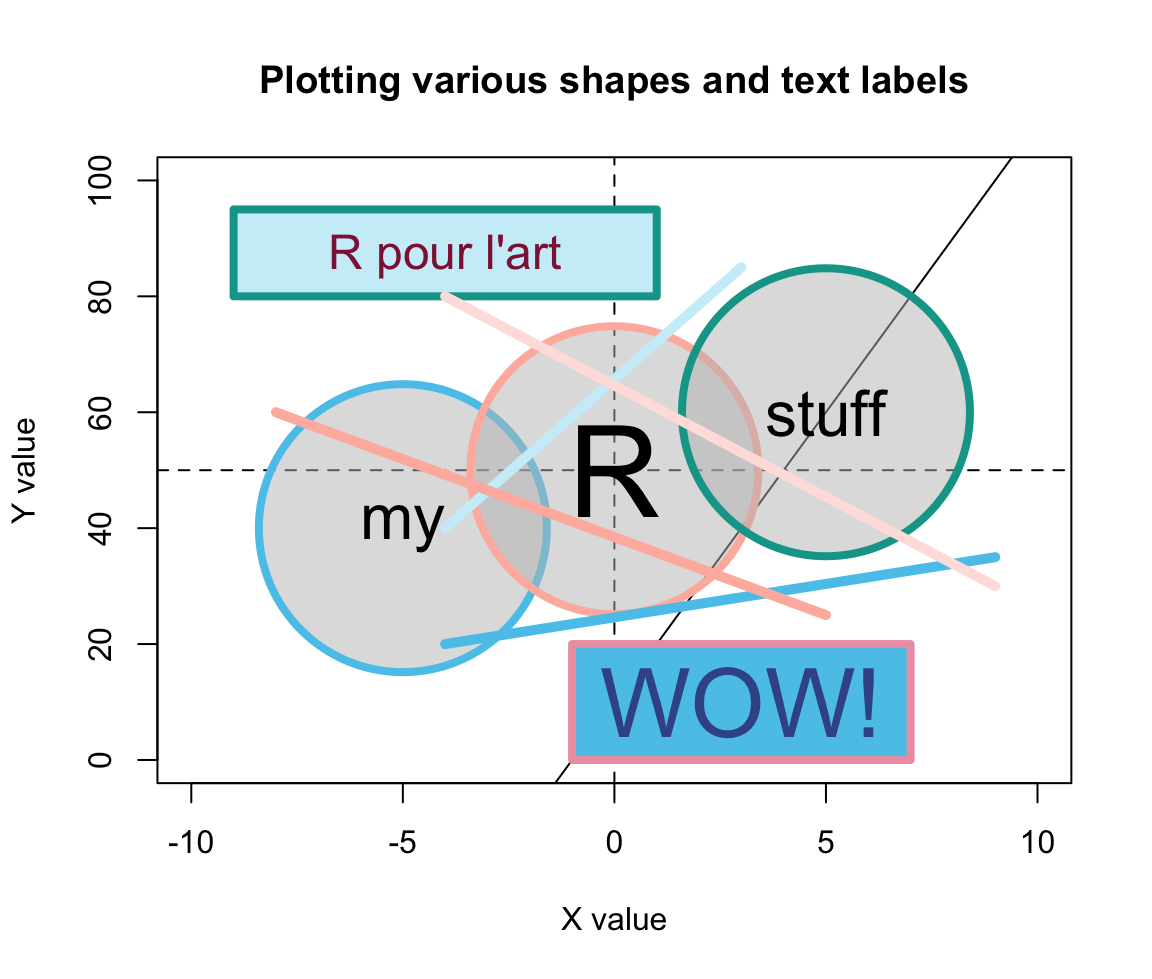Figure 4.5: An example of a complex plot, in which various elements are added to a prepared canvas.

Figure 4.5 shows that using transparent colors somewhat tempers the constraints imposed by sequentially plotting objects. For instance, the horizontal, vertical, and diagonal lines created by abline() (in Step 2) are not completely covered by the round shapes (due to setting alpha = 1/2 in their background color bg). By contrast, the two rectangles created by rect() (in Step 5) used a setting of alpha = 1 in their col definition. As a color’s alpha level ranges from 0 to 1 (with 0 indicating complete transprency and 1 indicating no transparency), using alpha = 1 is equivalent to “no transparency” — which is why the rectangles cover the lines created by abline().

Whereas Figure 4.5 arranges graphical elements without a particular purpose, the same methods can be used to produce highly versatile and useful visualizations. For instance, the R package riskyr package provides a range of visualizations that depict the effects of probabilistic binary distinctions based on a population of elements (aka. Bayesian reasoning or diagnostic testing).

Here is an example that illustrates the type of problem addressed by such diagrams:

• Suppose there is a pandemic (e.g., called COVID-19) that infects 5% of some population of people.

• There is a diagnostic screening test with the following properties:

• If someone is infected with COVID-19, the tests detects this accurately with a probability of 99%.

• If someone is not infected with COVID-19, the tests detects this accurately with a probability of 95%.

• Someone receives a positive test result. How likely is it that this individual is infected?

In diagnostic terms, the problem specifies the prevalence of some condition (here: 5% of some population are infected with COVID-19) and the sensitivity (99%) and specificity (95%) of some diagnostic test. The problem then asks for the conditional probability of being infected, given a positive test result (which is known as the test’s positive predictive value, PPV).

Research has shown that this problem is notoriously difficult, even for medical experts (see REFs for an analysis and meta-review). As the correct answer is the inverse conditional probability of the sensitivity provided (and we are also provided with a base rate/prevalence and the specificity), the answer could be computed using Bayes’ theorem.

ToDo: Bayes theorem: Show formula and result: PPV = 51%

Note: Given these values, the predictive value of a positive test result (PPV) is about 51%. Thus, throwing a coin is about as accurate as a single result of this test. (Importantly, this does not mean that the test is bad or useless. Things change dramatically if the prevalence of the condition in your environment (which is typically only a sub-part of the population) increased. For instance, if the prevalence of Covid in your environment was 20%, the same test would have a PPV of 83.2%. Alternatively, performing multiple tests with positive results would quickly raise the value of the test results.)

However, there are many other ways of computing the correct answer — and most of them involve a representational change in perspective (see Neth et al., 2021, for an analysis). Our goal here is not to solve the problem, but merely to illustrate that the problem could be solved by drawing frequency diagrams — and that we could use base R functions for creating relatively complex diagrams out of lines, shapes, and text labels. For many problems, we do not even need to do this ourselves, as the chances are quite high that someone else has already written an R package that does the job for us. In the current case, the riskyr package illustrates the relationship between a diagnostic test’s accuracy and predictive values in a variety of ways. (Note that you do not need to install the package for yourself, unless you wanted to re-create the plots.)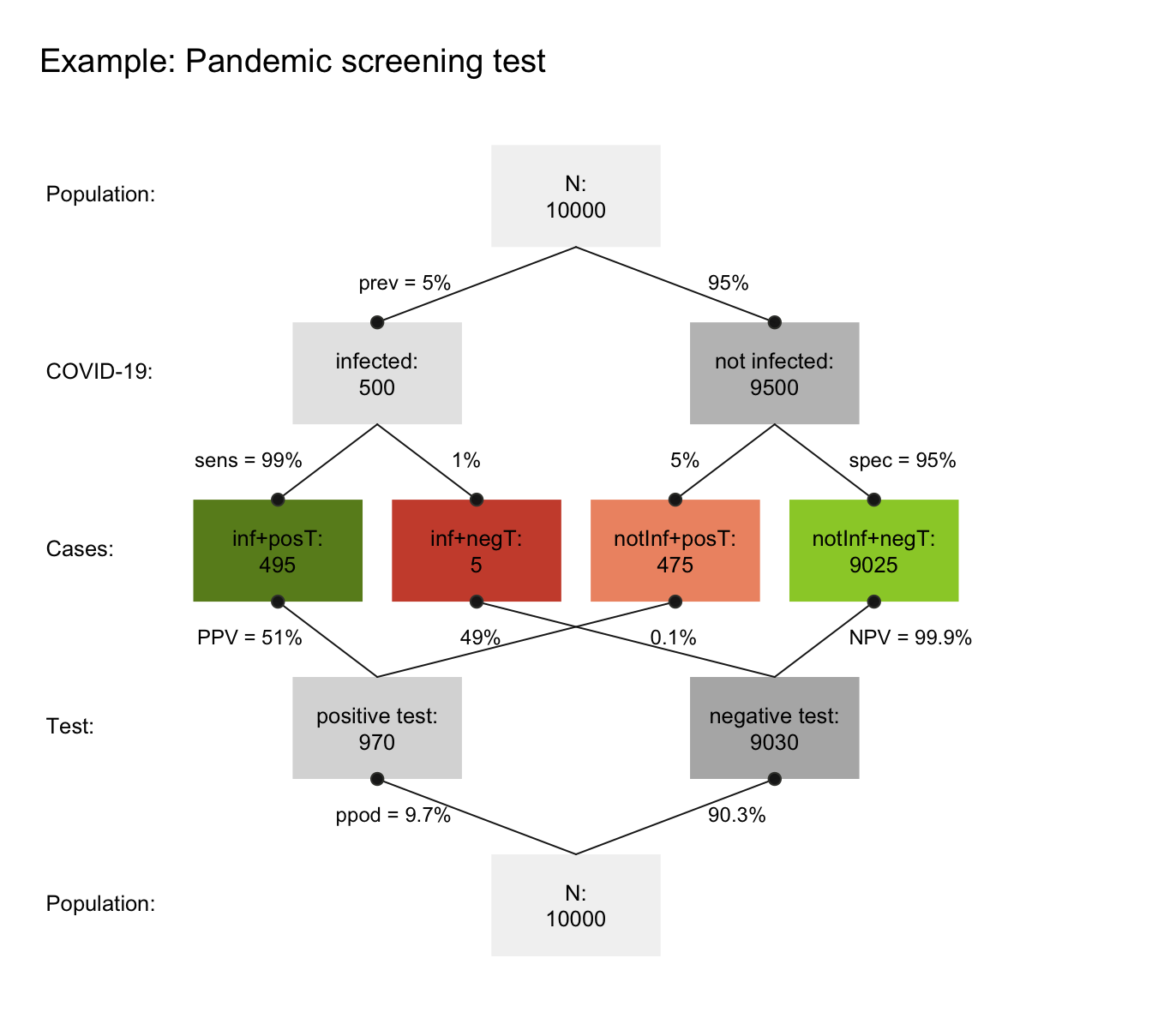Figure 4.6: A prism diagram illustrating the relation between diagnostic accuracy and predictive power of a test (using the riskyr package).

Figure 4.6 illustrates the relation between the test’s ability for detecting infected vs. healthy people and its predictive values in a diagram that combines two trees (one top-down, and an inverted one bottom-up). To provide concrete frequencies, both trees assume a fixed population of 10,000 individuals. The top one first dissects the population by condition (cd, i.e., 5% of the people are infected vs. 95% are not infected), then by the specificity and sensitivity of the test. The lower and inverted tree re-combines the four middle cells (in which green cells indicate correct classifications, whereas red ones indicate erroneous classifications) by test result or decision (dc, i.e., positive vs. negative tests). This reveals that the desired probability of being infected, given a positive test result is only $$PPV=51$$% — much lower than most people would expect.

Importantly, we could create visualizations like Figure 4.6 from scratch by plotting boxes, lines, and labels. As this would be a lengthy and laborious process, R packages like riskyr facilitate creating various types of plots (see riskyr.org for interactive versions). But it is good to know that we can always create things ourselves, if we had to or wanted to do so.

### 4.4.4 Useful combinations

In practice, most R plots require not just one command, but multiple functions. We typically start out with a simple plot and then add or change elements to improve it. Here are some combinations that are quite common and worth knowing:

#### Example 1

Example 1: A scatterplot with a grouping variable (and legend):

The iris data contained in datasets of R contains four types of measurements of three Species of flowers. Suppose we wanted to plot the relationship between Sepal.Length and Petal.Length as a scatterplot. The following plot would reveal a positive correlation between both measurements:

plot(x = iris$Sepal.Length, y = iris$Petal.Length,  # x and y variables
pch = 16, cex = 2,                             # aesthetic parameters
xlab = "Sepal Length", ylab = "Petal Length",  # axis labels
main = "Flower characteristics in Iris")       # title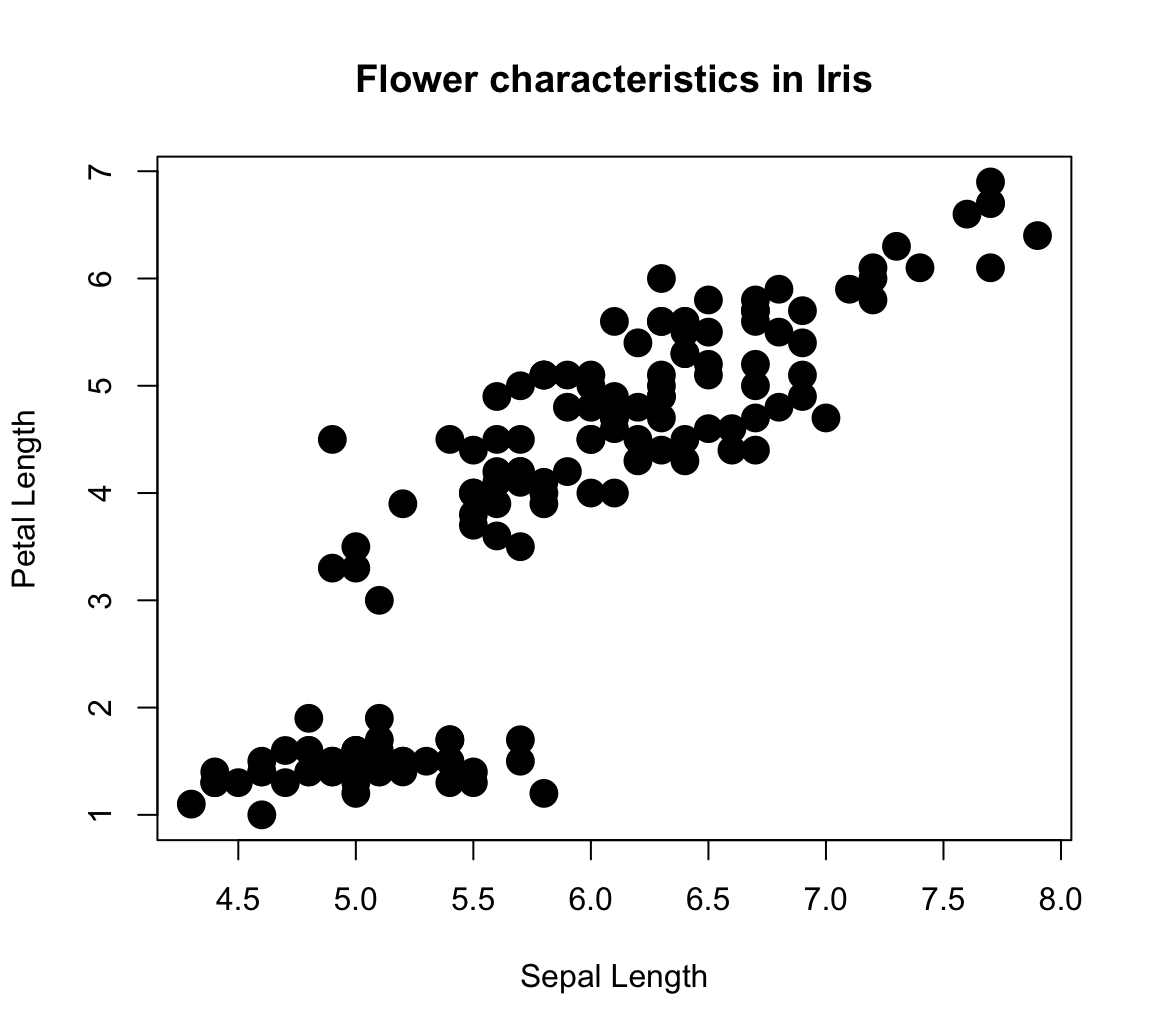However, the plot is deficient in an important respect: We lack all information regarding the Species of each point (which happens to be a factor variable of the iris data). An interesting feature of scatterplots is that we can assign its col aesthetic to a factor variable (i.e., internally encoded by a different integer value for each level of a categorical variable). The R plotting system uses a color vector palette() as its default colors. Setting col = iris$Species essentially instructs R to use the 1st color of palette() for the 1st species, the 2nd color of palette() for the 2nd species, etc. plot(x = iris$Sepal.Length, y = iris$Petal.Length, # x and y variables col = iris$Species,                            # color by species!
pch = 16, cex = 2,                             # aesthetic parameters
xlab = "Sepal Length", ylab = "Petal Length",  # axis labels
main = "Flower characteristics in Iris")       # title

grid()

legend (x = 4.5, y = 7, legend = levels(iris$Species), pch = 16, col = c(1:3))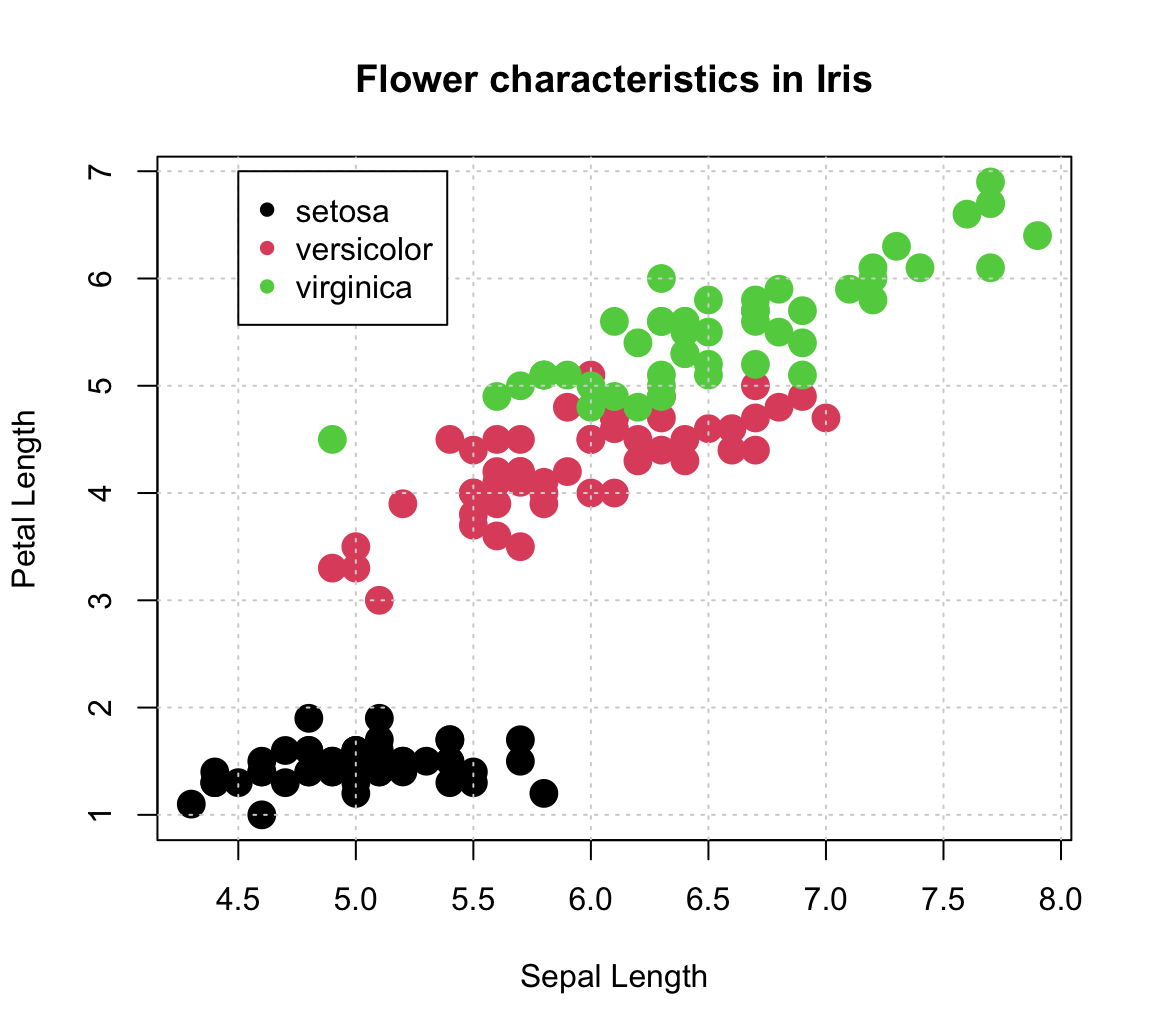Note that we used the legend() function to explicate the mapping of colors to species. The function’s x- and y-coordinates placed its top-left corner inside the plot, but its two key arguments were: • legend to print the levels of iris$Species (as a character vector);

• col explicitly set to the first three colors of the default palette().

Defining another color palette:

# (a) Default (base R):
palette()  # show current default colors
#>  "black"   "#DF536B" "#61D04F" "#2297E6" "#28E2E5" "#CD0BBC" "#F5C710" "gray62"

# Set to different values:
palette(rainbow(4))  # use color function
palette(c("steelblue", "gold", "firebrick", "forestgreen"))  # use color() names

# (b) Use hcl color palettes:
# hcl.pals()
palette(hcl.colors(3, "Red-Blue"))
palette(hcl.colors(3, "Viridis"))

# (c) color packages:
library(unikn)
palette(usecol(pal_unikn_pref))       # use color function with palette
palette(c(Seeblau, Pinky, Seegruen))  # use color names
palette(usecol(c(Seeblau, Pinky, Seegruen), alpha = 2/3))  # by names, plus transparency

# palette("default")  # reset to default color palette

plot(x = iris$Sepal.Length, y = iris$Petal.Length,  # x and y variables
col = iris$Species, # color by species! pch = 16, cex = 2, # aesthetic parameters xlab = "Sepal Length", ylab = "Petal Length", # axis labels main = "Flower characteristics in Iris") # title # Adding grid: grid() # Adding a legend: legend (x = 4.5, y = 7, legend = levels(iris$Species),
pch = 16, col = c(1:3))

# Linear regression:
fit <- lm(Petal.Length ~ Sepal.Length, data = iris)   # carry out linear regression
# fit
# summary(fit)
abline(fit, lty = "dashed", col = Karpfenblau, lwd = 2)    # add regression line

text(x = 7, y = 6.7, col = Karpfenblau,
labels = "R^2 = .76\nP < 2.2e-16")   # add a label to the plot at (x,y)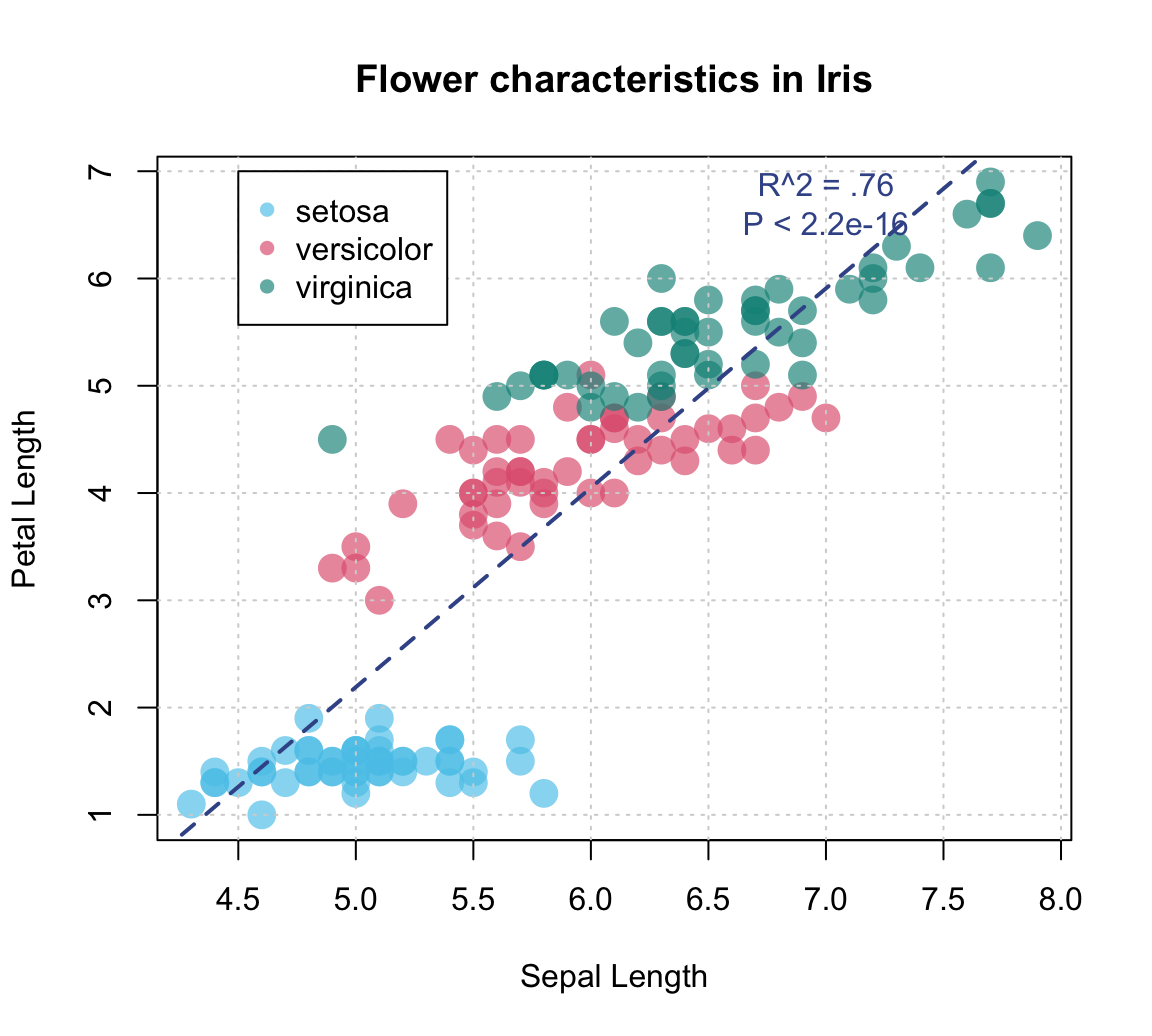An alternative (older) example:

# Demo: Basic scatterplot (based on some data) with a regression line:

# Create data:
year <- ds4psy::what_year(ds4psy::sample_date(from = "1990-01-01", to = "2002-12-31", size = 100),
as_integer = TRUE)
value <- 2 * (year - 1990) + runif(length(year), 0, 100)
df <- data.frame(year, value)

# Scatterplot:
plot(df$year, df$value, type = "p",
col = "skyblue", lwd = 2,                     # aesthetic parameters
xlab = "X label", ylab = "Y label",       # axis labels
main = "Line plot with regression line")  # plot title

# Regression:
fit <- lm(value ~ year, data = df)           # carry out linear regression
fit
#>
#> Call:
#> lm(formula = value ~ year, data = df)
#>
#> Coefficients:
#> (Intercept)         year
#>   -2410.625        1.238
abline(fit, lty = "dashed", col = "blue", lwd = 2)    # add regression line

summary(fit)
#>
#> Call:
#> lm(formula = value ~ year, data = df)
#>
#> Residuals:
#>     Min      1Q  Median      3Q     Max
#> -49.707 -20.278  -0.705  24.112  51.957
#>
#> Coefficients:
#>               Estimate Std. Error t value Pr(>|t|)
#> (Intercept) -2410.6248  1479.2834   -1.63   0.1064
#> year            1.2378     0.7412    1.67   0.0981 .
#> ---
#> Signif. codes:  0 '***' 0.001 '**' 0.01 '*' 0.05 '.' 0.1 ' ' 1
#>
#> Residual standard error: 26.39 on 98 degrees of freedom
#> Multiple R-squared:  0.02767,    Adjusted R-squared:  0.01775
#> F-statistic: 2.789 on 1 and 98 DF,  p-value: 0.09811
text(x = 2000, y = 20,
labels = "R^2 = 0.123\nP = 1.2e-12")   # add a label to the plot at (x,y)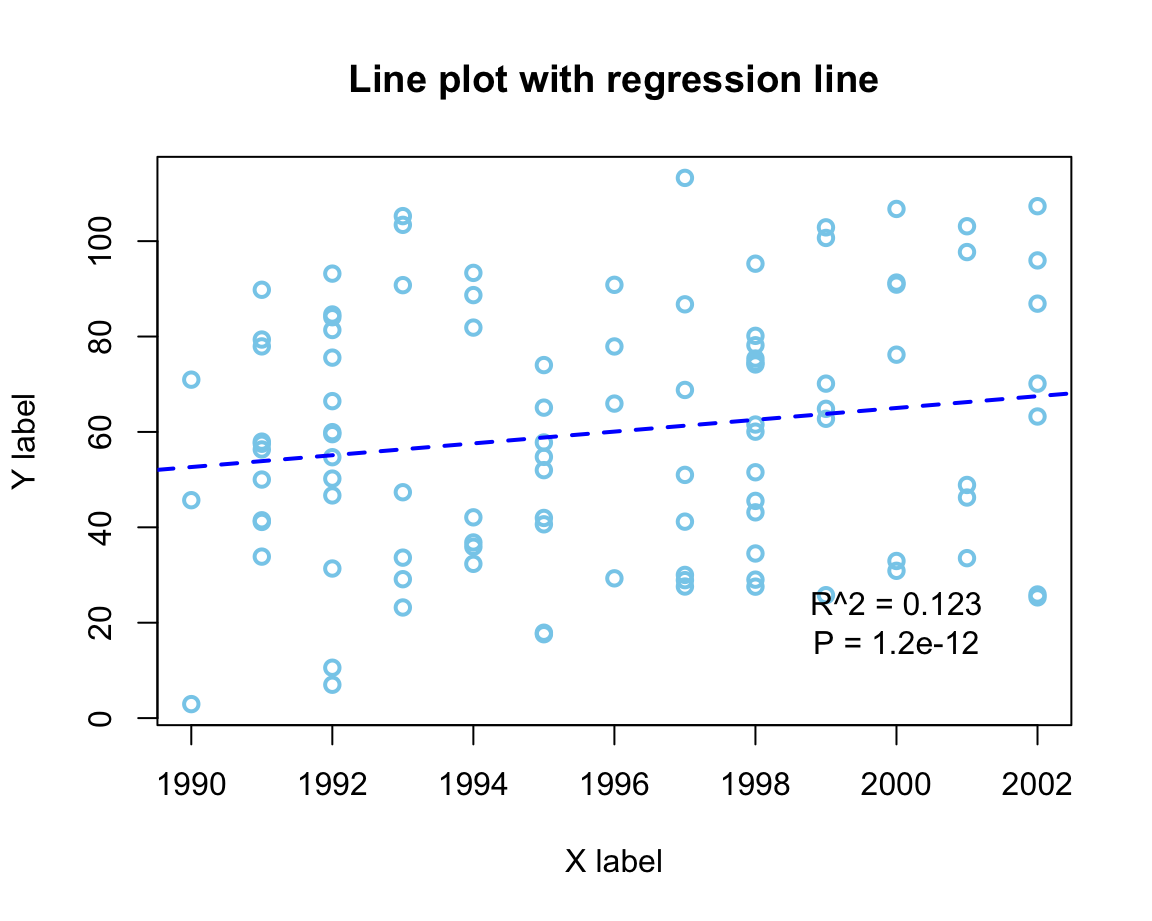#### Example 2

Example 2: Plotting grouped raw data as a box plot:

A scatterplot makes sense when both the x- and the y-dimension can be mapped to continuous variables. If one variable is continuous, but another one is categorical, the plot() function automatically chooses a box plot as a better alternative (see Figure 4.3). For instance, when using the mpg data to plot the values of the continuous variable cty as a function of the categorical variable class, we get:

plot(cty ~ class, data = mpg,
pch = 20, cex = 2,
col = usecol(Seegruen, alpha = 1/4),
main = "Fuel consumption in city by class of car")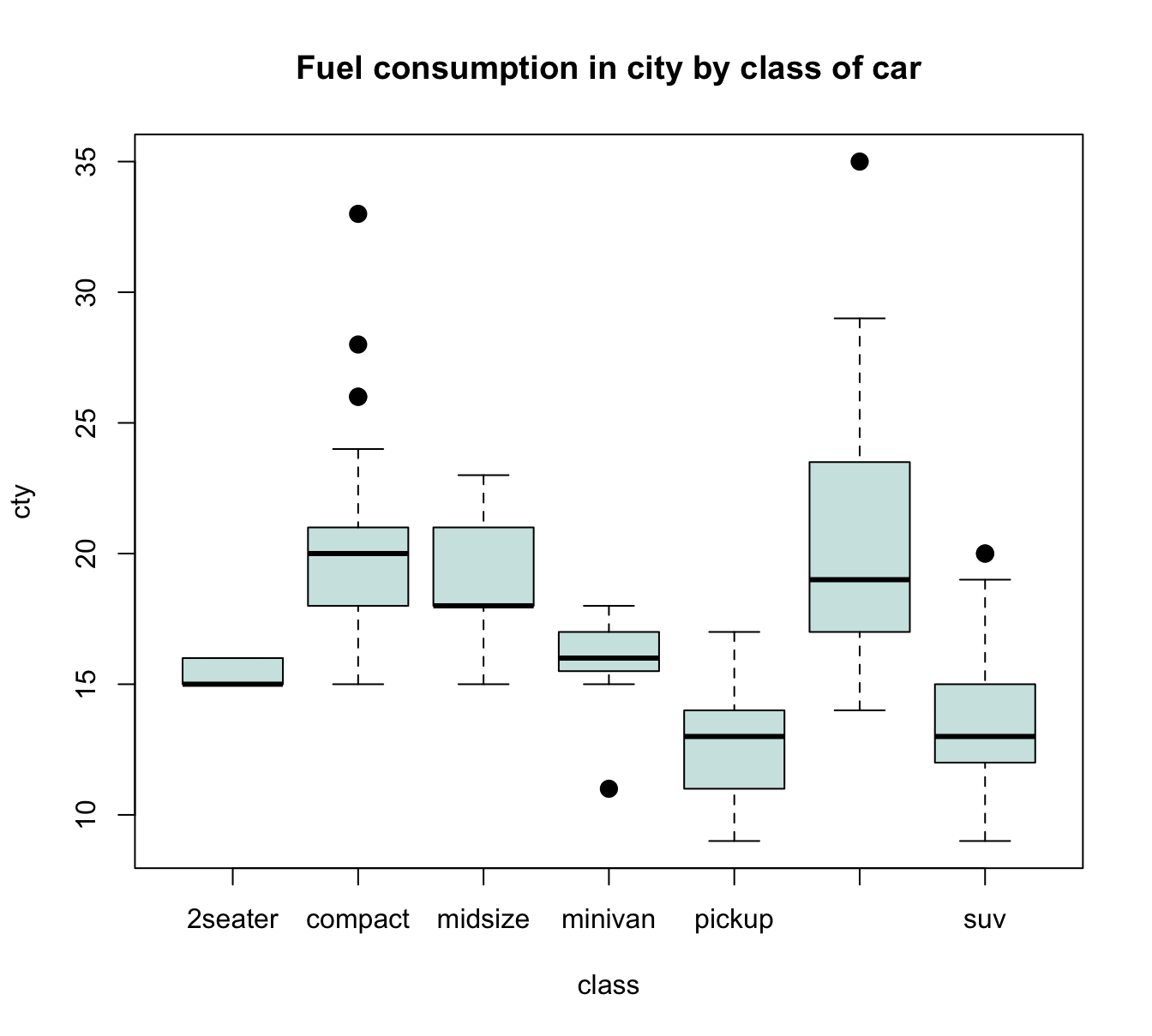Figure 4.7: A boxplot showing a continuous y-variable as a function of a categorical x-variable.

Alternatively, it often is desirable to also see the individual data points. A means of doing so is provided by the jitter(x, factor) function, that accepts a numeric vector x and a factor or amount by which the values of the numeric vector are to be jittered (i.e., randomly increased and decreased). The idea behind jittering is that adding random noise can sometimes help to distinguish between elements (e.g., when plotting them). Jittering works best in combination with transparent colors, as this enables us to see the density of overlapping elements.

In our present context, we primarily would need to add noise to our x-variable class, so that its values become distinguishable. Using jitter(as.numeric(class), 3/4) as our x-variable automatically transforms the boxplot into a scatterplot. However, we could also add some noise to our y-variable cty, which reduces the amount of overlap of points. Here are the results of both plots:

plot(cty ~ jitter(as.numeric(class), 3/4), data = mpg,
pch = 20, cex = 2,
col = usecol(Bordeaux, alpha = 1/4),
main = "A. cty by class (with horizontal jitter)")

plot(jitter(cty, amount = 3/4) ~ jitter(as.numeric(class), 3/4), data = mpg,
pch = 20, cex = 2,
col = usecol(Bordeaux, alpha = 1/4),
main = "B. cty by class (with horizontal and vertical jitter)")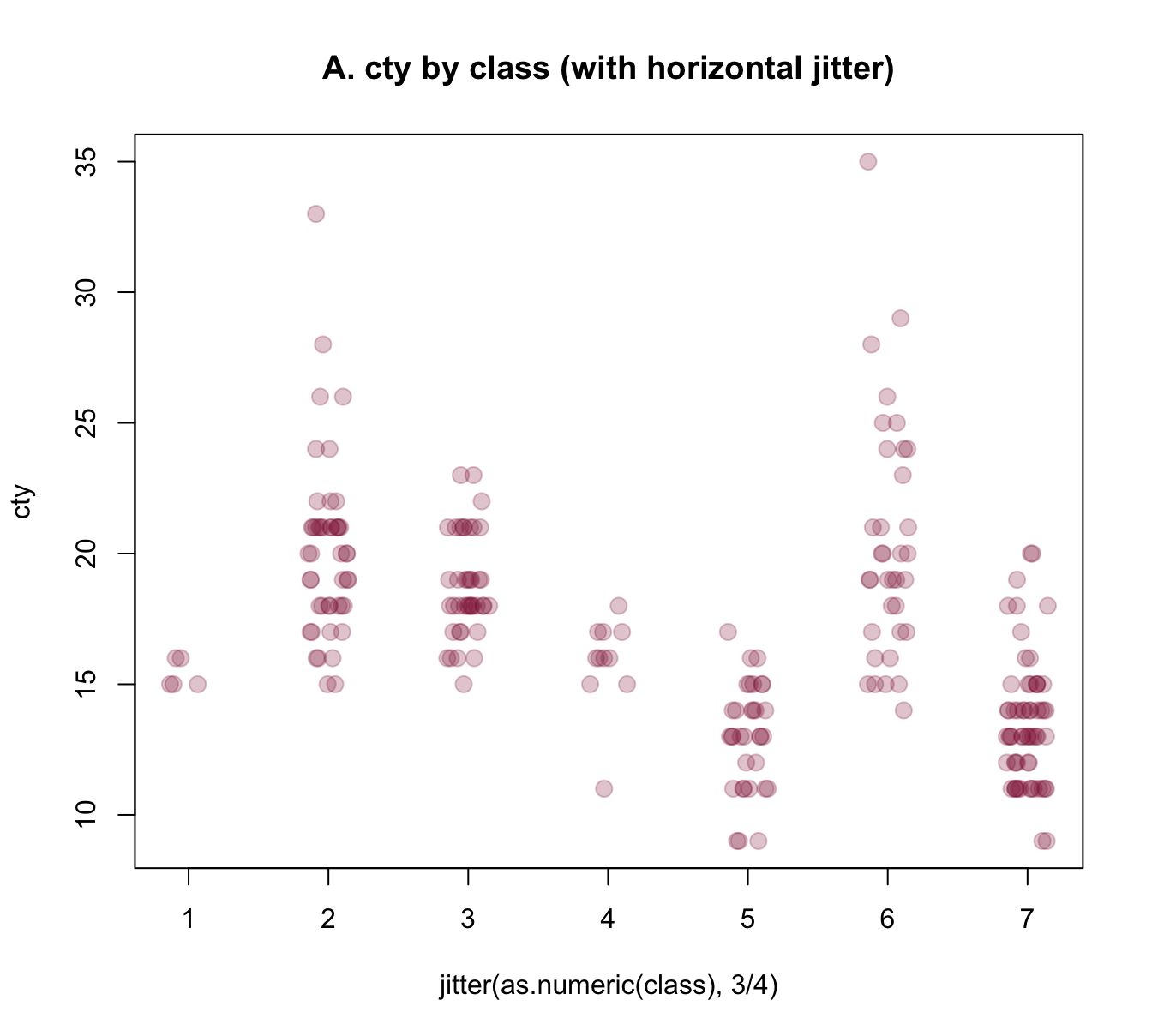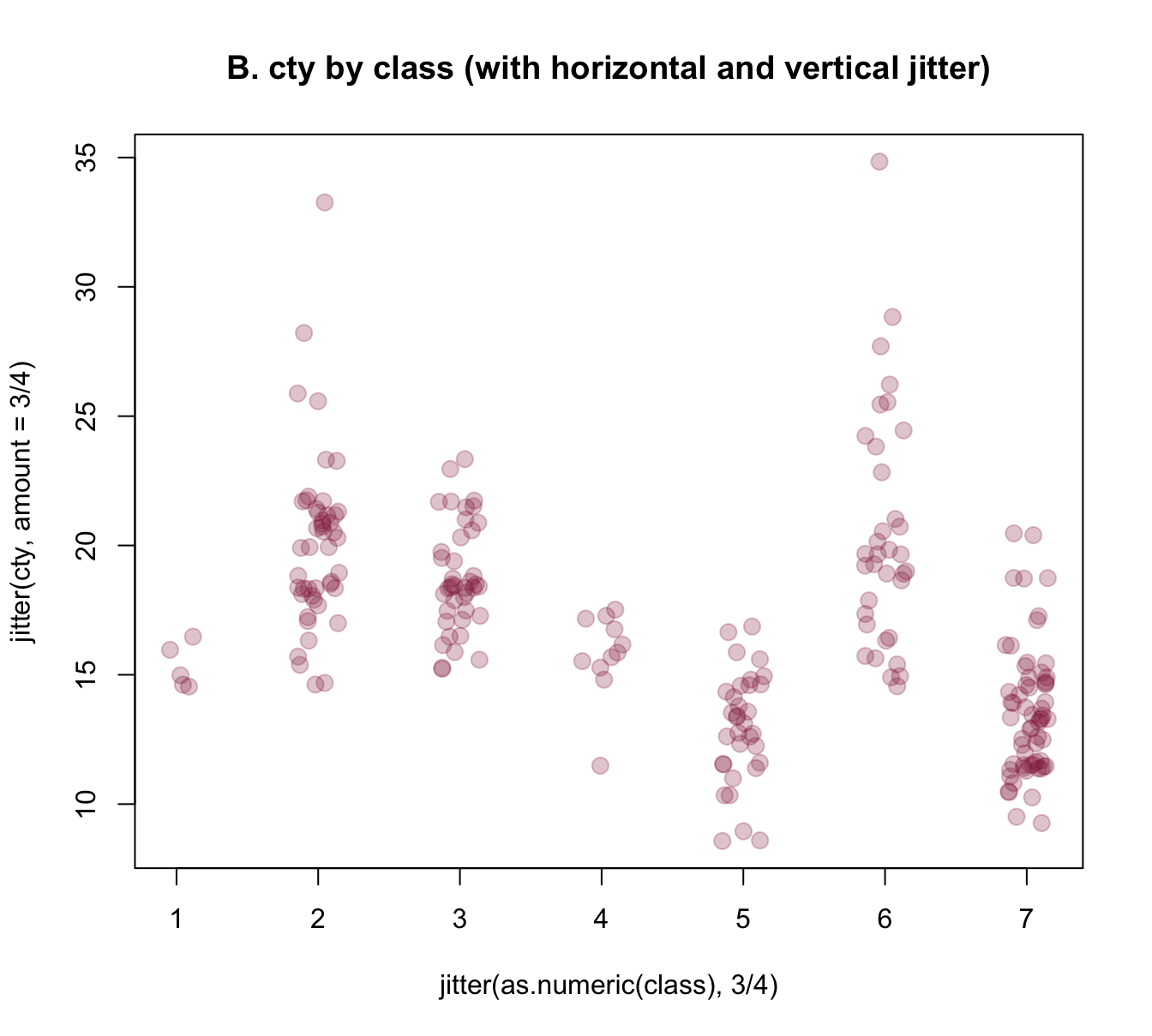Figure 4.8: Jittering categorical or/and continuous variables to show raw data points as scatterplots.

The jittered raw data plots of Figure 4.8 provide some additional information over the boxplot of Figure 4.7 (e.g., they nicely illustrate the group sizes), but also have some deficiencies:

1. As we converted class into a numeric variable, their x-axis is numeric, rather than categorical. Can we use the previous class labels on our x-axis?

2. The raw data plots lack the explicit information about means and dispersion of groups that the boxplot provided. Can we combine both plots to provide a more complete picture?

The answer to both questions is yes, of course. R provides several approaches for tackling these challenges, but all involve creating a plot in several steps.

To gain more control about the axes, we could use an initial plot() function to define the basic dimensions and labels of our plot, but not yet include any axes (by setting axes = FALSE). We then add two axis() commands (to explicitly define an x- and y-axis and specify its steps, labels, and the orientation of labels) and a grid() command (to show some orientation lines in the background). Finally, we can use the points() annotation function with the jitter() instructions from above to add our raw data points to the canvas. Overall, the resulting plot provides a better impression of the raw data points:

plot(x = 0, type = "n",
xlim = c(0.5, 7), ylim = c(0, 40),
axes = FALSE,
main = "Raw data plot (with random jitter)",
xlab = "Class X", ylab = "Value of Y")

axis(1, at = 1:7, labels = levels(mpg$class), las = 2) # x-axis axis(2, at = seq(0, 40, by = 5), las = 1) # y-axis grid() # add grid lines # plot raw data (as points with jitter): points(jitter(cty, 3/4) ~ jitter(as.numeric(class), 3/4), data = mpg, pch = 20, cex = 2, col = usecol(Bordeaux, alpha = 1/4))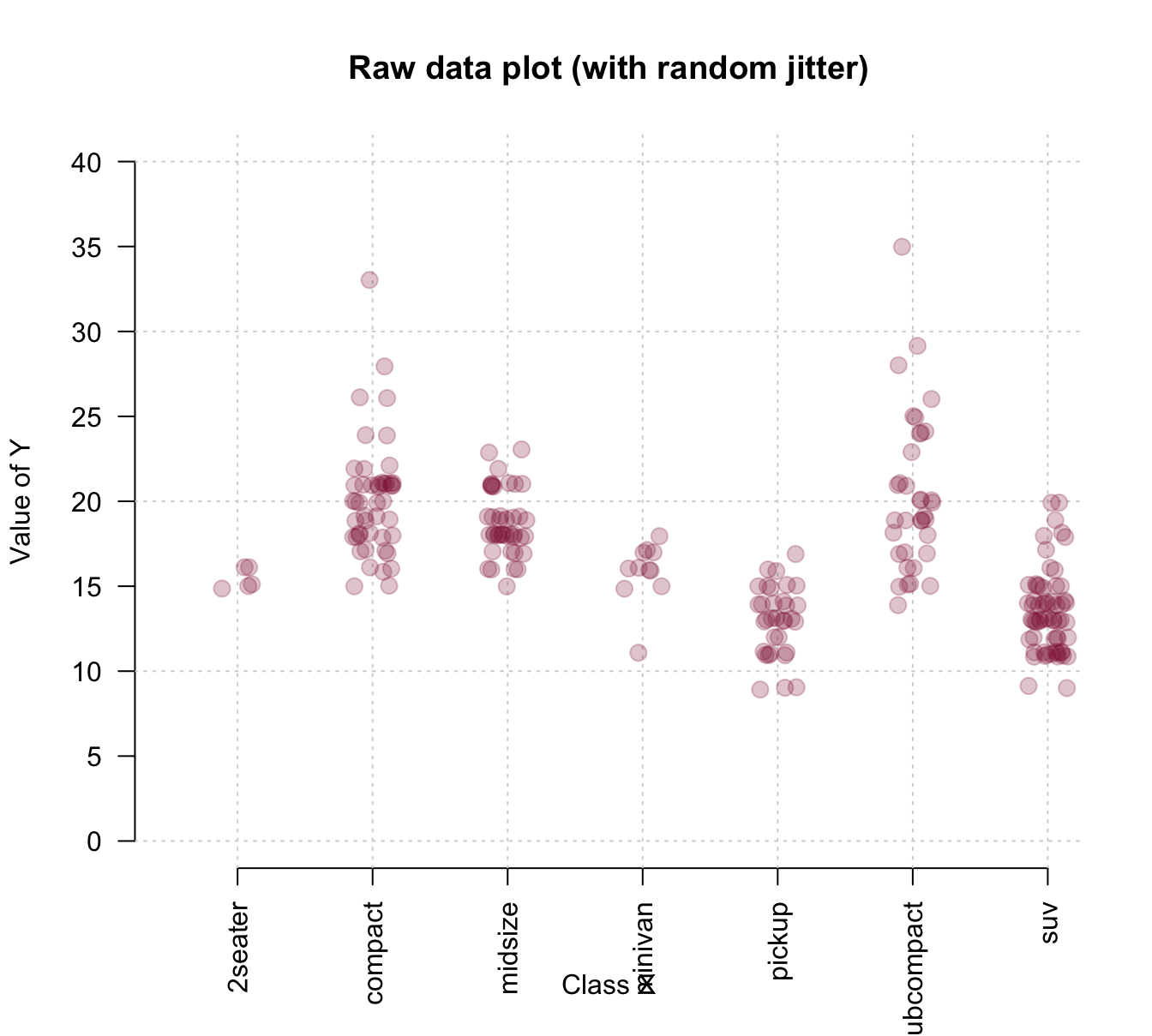To combine the benefits of the more abstract box plot with those of plotting the raw data, we could first draw a boxplot and later add the individual data points to it. Saving the boxplot to an R object also allows us to later use this object to add details to the plot. The following example inspects and uses the object bp to add text labels of group sizes and label two outliers: # boxplot: bp <- boxplot(cty ~ class, data = mpg, col = usecol(Seegruen, alpha = 1/4), las = 2, ylim = c(0, 40), main = "Boxplot (with jittered raw data and some details)", xlab = "Class of car", ylab = "MPG in city") grid() # add grid lines # plot raw data (as points with jitter): points(jitter(cty, 3/4) ~ jitter(as.numeric(class), 3/4), data = mpg, pch = 20, cex = 2, col = usecol(Bordeaux, alpha = 1/4)) # Add group sizes (as text labels): text(x = 1:7, y = 0, labels = paste0("[", bp$n, "]"), cex = .85)

## Check some outliers:
# bp$out # bp$group
# mpg[mpg$cty>30, ] # Add some text labels: text(x = 2, y = bp$out, labels = "VW New Beetle 1999",
cex = .85, col = Bordeaux, pos = 3)
text(x = 6, y = bp$out, labels = "VW Jetta 1999", cex = .85, col = Bordeaux, pos = 3)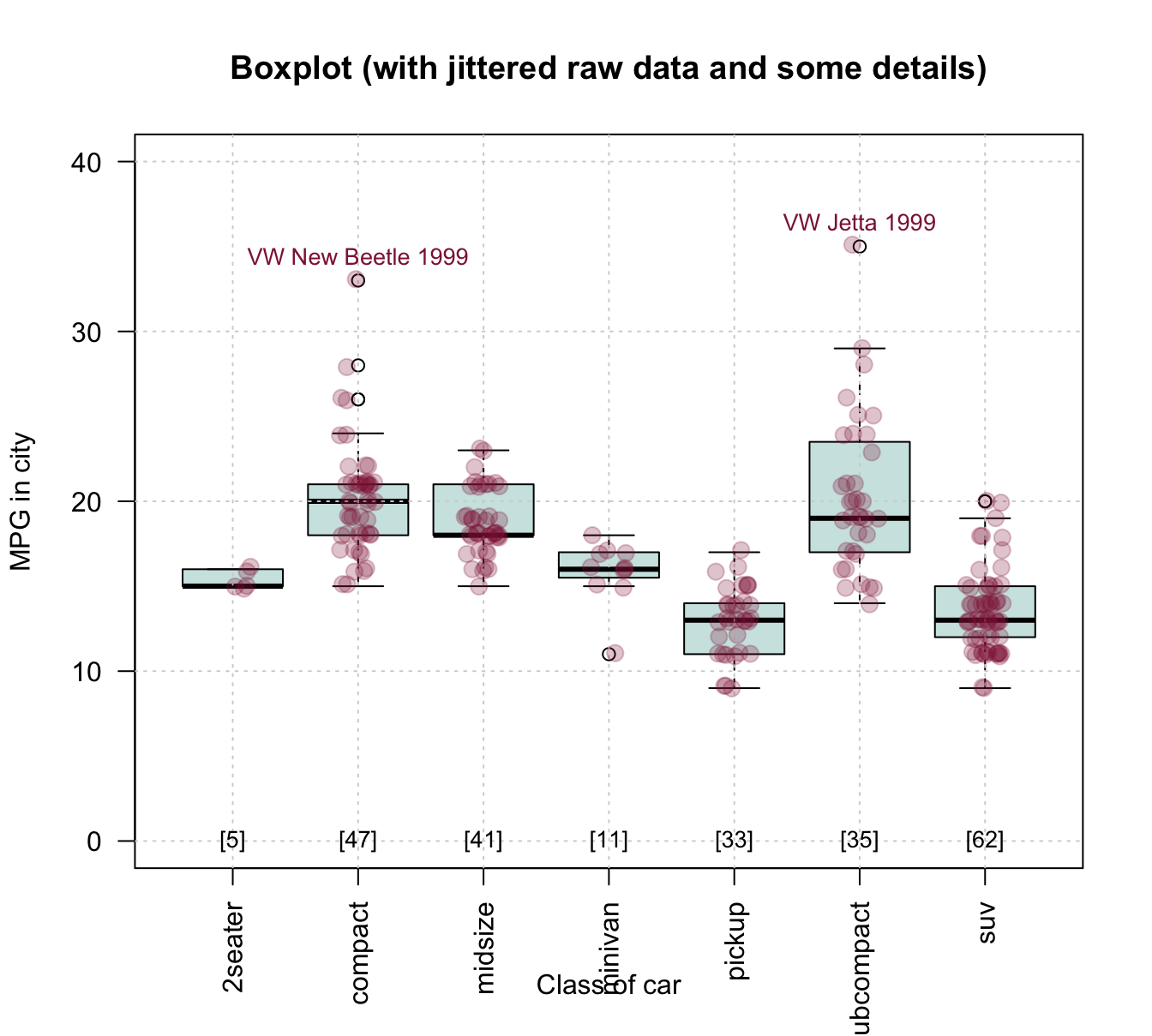Figure 4.9: Combining a box plot and a jittered raw data plot (plus some details). #### Example 3 Example 3: Plotting curves from functions: Given some diagnostic test’s sens and spec values, what are the test’s positive and negative predictive values (PPV and NPV) as a function of prev? Without understanding any details, we can use the functions provided by some package to compute the desired values. Here, we use the riskyr package (mentioned above, but not needed to create the visualizations of this section): library(riskyr) comp_PPV(prev = .05, sens = .99, spec = .95) #>  0.5103093 comp_NPV(prev = .05, sens = .99, spec = .95) #>  0.9994463 However, rather than just making predictions for individual prevalence values prev, we want a curve that shows PPV and NPV as a function of prev: # Prepare canvas: plot(x = 0, type = "n", xlim = c(0, 1), ylim = c(0, 1), main = "Predictive values as a function of prevalence", xlab = "Prevalence", ylab = "Predictive values") grid() # Set parameters and compute some points: prev <- .05 PPV <- comp_PPV(prev = prev, sens = .99, spec = .95) NPV <- comp_NPV(prev = prev, sens = .99, spec = .95) col_PPV <- "darkorange" col_NPV <- "steelblue" # Curves: curve(expr = comp_PPV(prev = x, sens = .99, spec = .95), from = 0, to = 1, col = col_PPV, lwd = 2, add = TRUE) curve(expr = comp_NPV(prev = x, sens = .99, spec = .95), from = 0, to = 1, col = col_NPV, lwd = 2, add = TRUE) # Vertical line: abline(v = prev, lty = 2, lwd = 1, col = "grey50") # Points: points(x = prev, y = PPV, pch = 20, cex = 2, col = col_PPV) points(x = prev, y = NPV, pch = 20, cex = 2, col = col_NPV) # Text labels: text(x = prev, y = PPV, label = "PPV", cex = 1, pos = 4, col = col_PPV) text(x = prev, y = NPV, label = "NPV", cex = 1, pos = 1, col = col_NPV)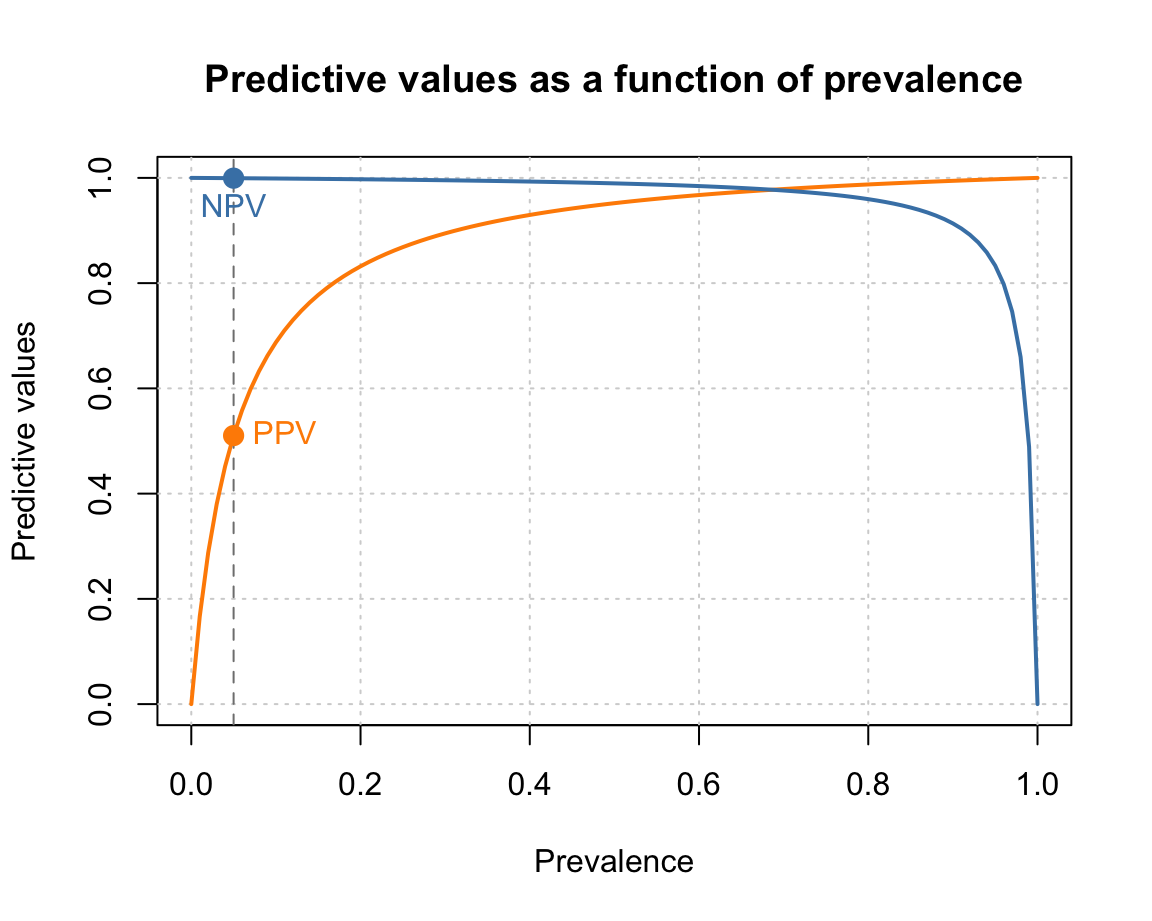Note that creating such plots implies many organizational and structural aspects. For instance, we first define some constants so that they can be used in various commands later. Similarly, the order of functions matters, as objects are plotted on top of earlier ones. Overall, designing such plots implies writing a small script or computer program. Each step either defines some feature or computes an object by applying a function. When the steps are arranged and evaluated in the right order, they create a graphical representation. ### 4.4.5 Setting graphical parameters (par()) The appearance of any graphical device in R depends on many graphical parameters. For instance, the dimensions and margins of plots, the colors of fore- or background elements, and the appearance of labels, points, or lines is governed by such parameters. The par() function allows both checking and setting these parameters. It displays and allows to change a large number of parameters as tagged values (i.e., in a form tag = value, where tag is the name of a graphical parameter and value its current or desired value). In this section, we introduce some important parameters (see ?par for the full list of parameters and options). #### Example parameters Here are the default values of the most important graphical parameters: # Plot margins par("mar", "oma") # margin (in lines on bottom, left, top, right) and outer margins #>$mar
#>  5.1 4.1 4.1 2.1
#>
#> $oma #>  0 0 0 0 # Colors: par("col", "bg") # color of plot foreground and background #>$col
#>  "black"
#>
#> $bg #>  "white" par("col.axis", "col.lab", "col.main") # additional colors #>$col.axis
#>  "black"
#>
#> $col.lab #>  "black" #> #>$col.main
#>  "black"

# Points:
par("pch")  # point symbol (0-25, see ?points() for values)
#>  1

# Lines:
par("lty", "lwd")  # line type and width
#> $lty #>  "solid" #> #>$lwd
#>  1

# Fonts:
par("family", "font")  # font family and type
#> $family #>  "" #> #>$font
#>  1

# Text and symbol size:
par("cex")  # magnification value for text and symbols
#>  1

See ?par for details and options.

#### Arranging multiple plots

We often want to fit two or more plots into the plotting area. The “mfrow” and “mfcol” parameters of par() provide or allow to change a vector c(nr, nc) that indicates the number of plots per row (nr) and column (nc). Thus, setting par(mfrow = c(1, 2)) would fit two plots into one row, whereas par(mfrow = c(2, 1)) would stack two plots on top of each other.

#### Storing parameters

Whenever changing the default graphical parameters, it is a good idea to store the original (or current) values, so that they can be restored later. This can easily be done by copying the current parameters in an object (e.g., in an R object opar, short for “original par”):

• opar <- par() stores the original (default) par settings

• par(opar) restores the original (default) par settings

As some parameters can only be read (but not changed), we typically exclude “read-only” parameters by storing opar <- par(no.readonly = TRUE). Here is an example for storing the current parameters, changing the defaults to create a plot, and then restoring the original parameters:

# 1. Store original parameters: ----

# 2. Change plotting parameters: ----

# (a) Arrange multiple plots:
par(mfrow = c(1, 2))  # 1 row, 2 columns

# (b) Change default parameters:
par(mar = c(4, 4, 2, 1),
bty = "l", pch = 20, lwd = 2,
family = "mono", font = 2, cex = .8,
col = "white", bg = "steelblue4",
col.main = "white", col.axis = "gold", col.lab = "orange")

# Plot plots (with these settings):
plot(x = mpg$cty, y = mpg$hwy, main = "A. mpg data")
plot(Nile, main = "B. Nile data")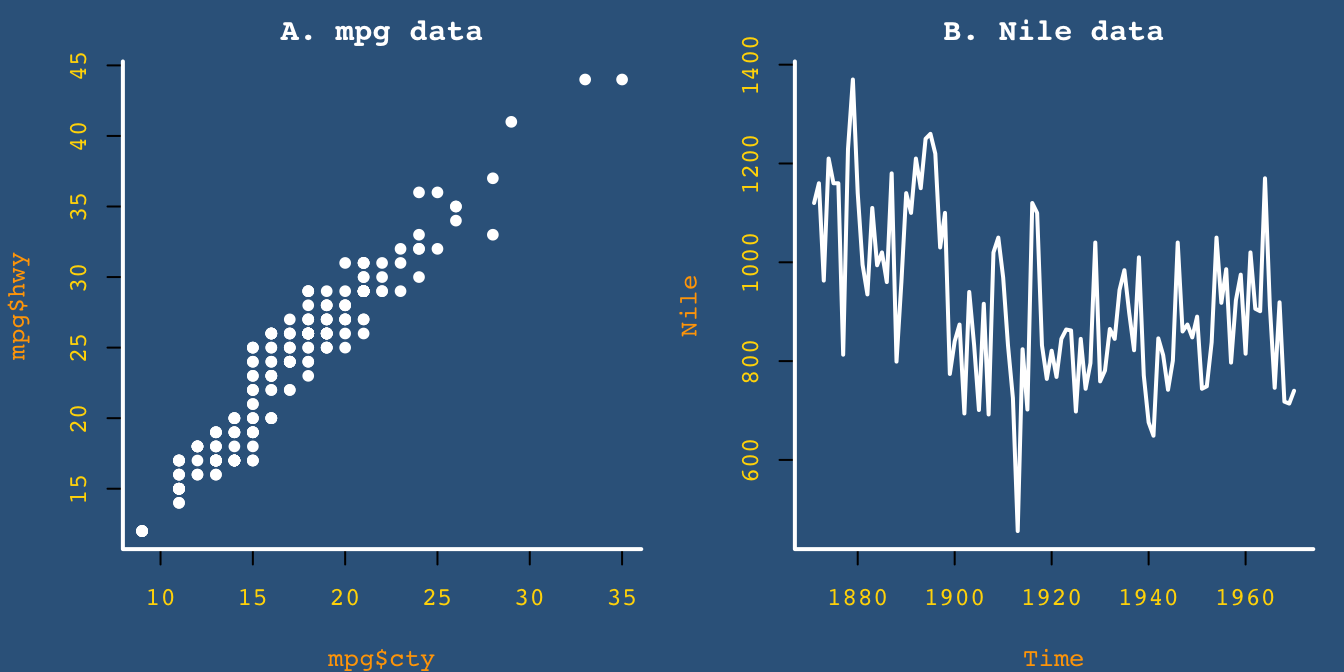# 3. Restore original parameters: ----
par(opar)

See ?par for the list of parameters and options.

#### Practice

1. The data frame in datasets::Orange provides the age and circumference values of five orange trees. Create a line plot that show their growth (i.e., circumference) as a function of their age.

Hint: Here’s what our result could look like: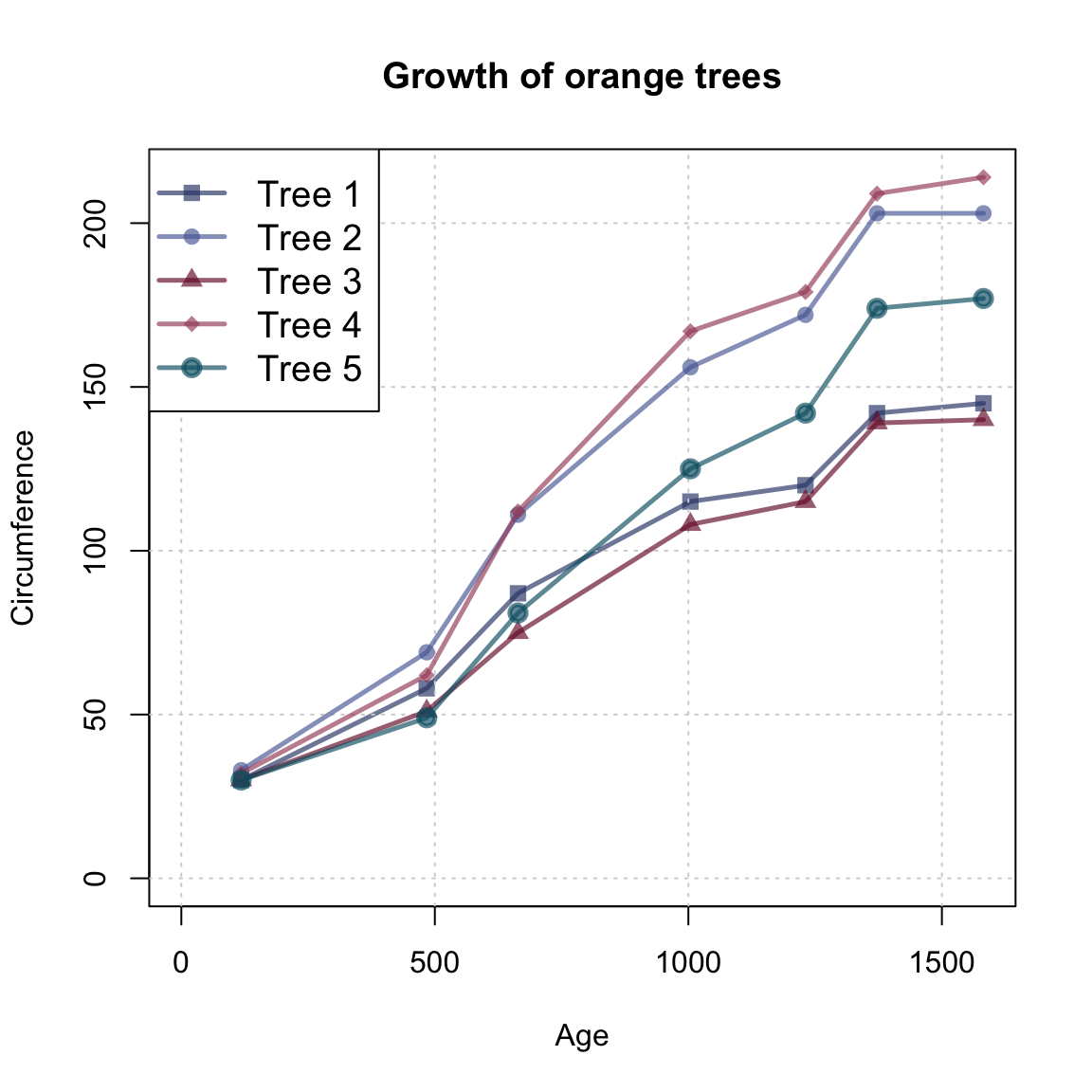#### Solution

We first copy the data into an object otree and inspect it:

# data:
otrees <- datasets::Orange
dim(otrees)
#>  35  3

# as tibble:
(tibble::as_tibble(otrees))
#> # A tibble: 35 × 3
#>   Tree    age circumference
#>   <ord> <dbl>         <dbl>
#> 1 1       118            30
#> 2 1       484            58
#> 3 1       664            87
#> 4 1      1004           115
#> 5 1      1231           120
#> 6 1      1372           142
#> # … with 29 more rows

table(otrees$Tree) # 7 rows/measurements per tree #> #> 3 1 5 2 4 #> 7 7 7 7 7 Note that the data provides measurements (of age and circumference) for 5 trees and contains a block of 7 measurements (rows) per tree. Step by step: library(unikn) # Define visual parameters: my_lwd <- 2.5 my_cex <- 1.2 my_col <- unikn::usecol(pal_unikn_dark, alpha = 2/3) # Basic plot (with first line): plot(x = otrees$age[1:7], y = otrees$circumference[1:7], type = "o", lwd = my_lwd, pch = 15, cex = my_cex, col = my_col, xlim = c(0, max(otrees$age)), ylim = c(0, max(otrees$circumference)), xlab = "Age", ylab = "Circumference", main = "Growth of orange trees") # Add grid: grid() # Loop through data frame (in 5 steps, reading 7 lines at a time): for (i in 1:5){ lines(x = otrees$age[((7*i) + 1):((7*i) + 7)],
y = otrees$circumference[((7*i) + 1):((7*i) + 7)], type = "o", lwd = my_lwd, pch = (15 + i), cex = my_cex, col = my_col[i + 1]) } # Adding legend: legend("topleft", legend = paste0("Tree ", 1:5), lwd = my_lwd, pch = 15:20, cex = my_cex, col = my_col)Note that the lines() function is embedded in a for loop (to generate multiple lines) and uses numeric indexing to select the block of 7 measurements (rows) from the otrees data that describes each tree. Rather than using a for loop and numeric indexing, we could have used logical indexing to identify each tree and repeated the following steps for each tree: # Tree 2: i <- 2 lines(x = otrees$age[otrees$Tree == i], y = otrees$circumference[otrees\$Tree == i],
type = "o", lwd = my_lwd, pch = (15 + i), cex = my_cex,
col = my_cols[i])
1. Further examining graphical parameters:
• What kind of data structure is returned by par()?

• How would a plot change when the graphical parameters were set to par(mar = c(0, 0, 0, 0), omi = c(1, 1, 1, 1))?

• What would change if the graphical parameters were set to par(mfrow = c(2, 1))?

• Which graphical parameters can be read, but not set?

Hint: Study the documentation of par(), or try storing and re-setting par_org <- par(); par(par_org).

### References

Neth, H., Gaisbauer, F., Gradwohl, N., & Gaissmaier, W. (2021). riskyr: Rendering risk literacy more transparent. https://riskyr.org/, https://CRAN.R-project.org/package=riskyr